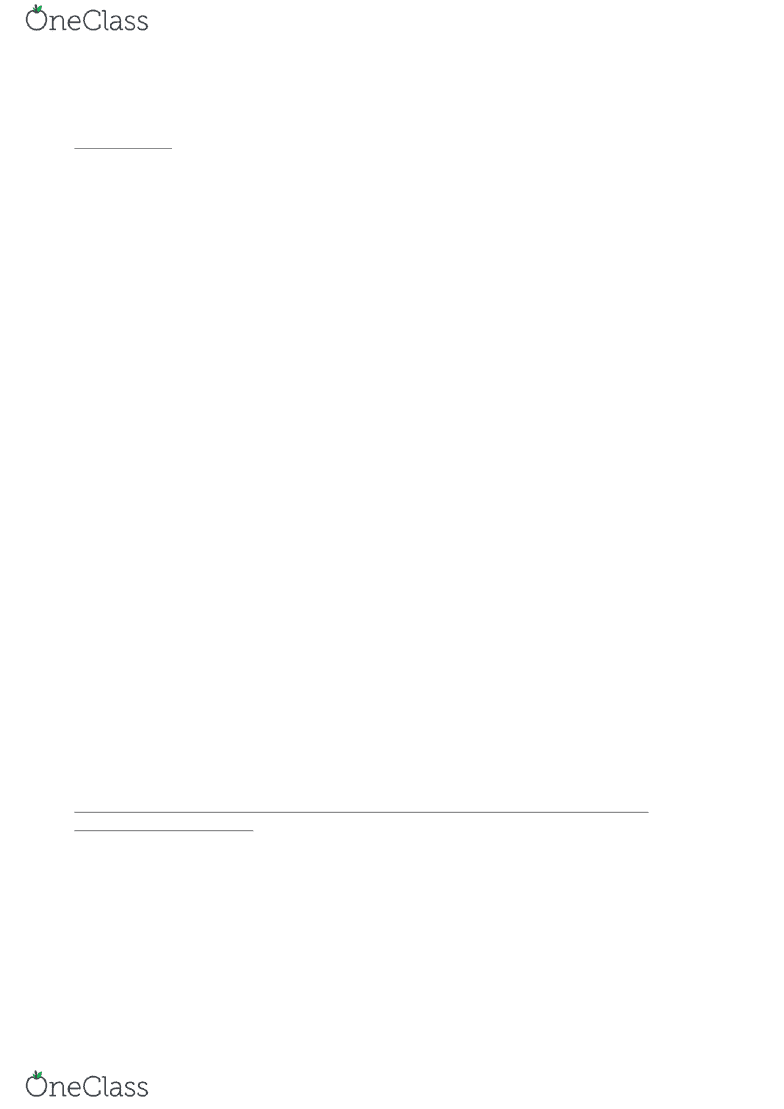# CHEM 122 Lecture Notes - Lecture 6: Ideal Gas Law, Reaction Rate, Wanda Cinemas

15 views3 pages
School
Department
Course

For unlimited access to Class Notes, a Class+ subscription is required.__________________________________________________________________________________________
CHAPTER 12
chemical kinetics, study of reaction rates
average rate: -D[Conc]/Dt
instantaneous rate= rate for speciﬁc instance in time (won’t be asked to calculate)
initial rate:
2C -> 1D
then rate = 2/2 x - D[Conc]/Dt
rate = 1/2 x + D[Conc]/Dt
If the rate of formation of NH3 is 2.7x10^2 M/s, what is the rate of disappearance of
Nitrogen and Hydrogen?
N2 + 3H2 -> 2NH3
1/2N2 + 3/2H2 -> 2/2NH3
N: rate= 1/2 (2.7x10^-2M/s)=1.4x10^-2M/s
H: rate=3/2 (2.7….
Factors that affect reaction rate
1. Temperature directly proportional
2. concentration or pressure of reactions directly proportional n/v=P/RT
….related to ideal gas law
3. Catalysts
4. Surface Area smaller SA increases Reaction Rate ….thats why gun powder
THE RATE LAW is determined experimentally and always deﬁned in terms of
reactant concentrations
aA +bB —> cC +dD this reaction is xth order in A
this reaction is yth order in B
Rate = k [A]^x[B]^y overall order = x+y *not related to the
stoichiometric coefﬁcient
k is rate constant, temp, dependent
Rate is M/time
find more resources at oneclass.com
find more resources at oneclass.com
Unlock document

This preview shows page 1 of the document.
Unlock all 3 pages and 3 million more documents.

Already have an account? Log in

# Get access

Grade+
\$10 USD/m
Billed \$120 USD annually
Homework Help
Class Notes
Textbook Notes
40 Verified Answers
Study Guides
1 Booster Class
Class+
\$8 USD/m
Billed \$96 USD annually
Homework Help
Class Notes
Textbook Notes
30 Verified Answers
Study Guides
1 Booster Class